### 4.6Effect of the MTJ Device Parameters on Reliability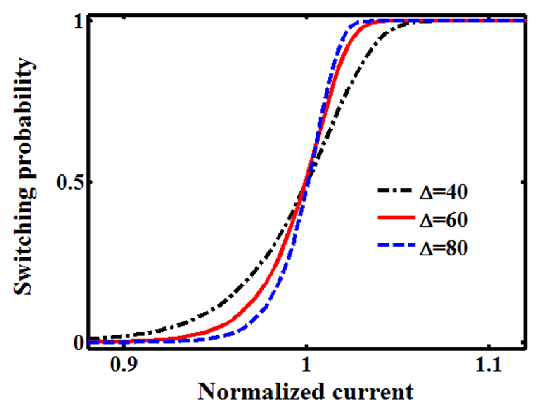Figure 4.23.: Switching dynamics of the MTJ device as function of the applied current plotted for different values of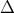.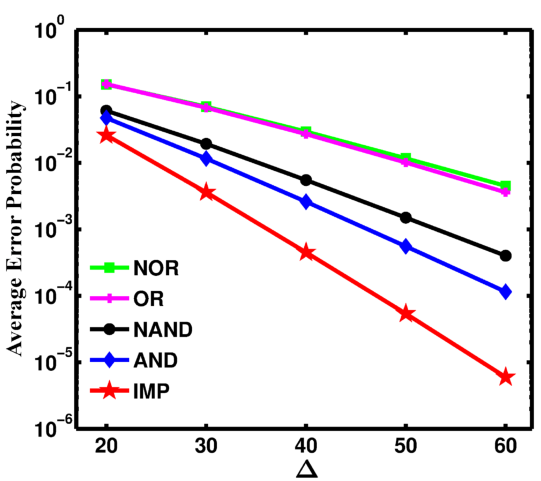Figure 4.24.: Average error probabilities for the basic operations of the two-input reprogrammable gate (AND, OR, NAND, and NOR) and the proposed CC-IMP gate (IMP) as a function of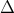for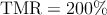.

As mentioned before, the TMR ratio is considered as a very important device parameter for the reliability of the conditional switching in MTJ-based logic gates. However, other MTJ device parameters also affect the reliability. Fig. 4.23 shows the switching probabilities of the MTJ device in the thermally-activated switching regime (Eq. 4.15) as a function the MTJ current for different values of the thermal stability factor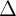. It illustrates that a higher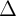provides sharper switching dynamics (switching windows shown in Fig. 4.19 and Fig. 4.13). As decreasing the SWs increases the width of the reliable gaps opened between the SWs of the desired and undesired switching events, we expect a more reliable logic behavior for a higher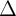. In fact, according to Eq. 4.15, the dominant term for the switching probability calculation is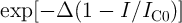. The modulation of the term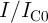depends on the TMR ratio and its impact has been studied before. Nevertheless, a highermagnifies the effect of this modulation. Therefore, for all MTJ-based logic operations, a higher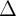decreases the error probabilities. Indeed, Fig. 4.24 shows the average error probabilities of the basic operations of the CC-IMP and the two-input reprogrammable gates as a function of the thermal stability factor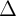. It illustrates that the error decreases exponentially with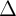and for the same device characteristics and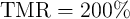, the CC-IMP gate exhibits always a more reliable logic behavior as compared to the two-input reprogrammable gate. At each point the circuit parameters (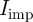and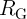in Fig. 4.15b and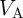in Fig. 4.20) are optimized to minimize the error probability. As it is shown in , although a lower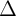value allows easier STT switching, a minimum value of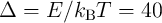is required for low write and read error rates in STT-MRAM technology. A similar trade-off is encountered in the design of the MTJ-based logic gates. According to Eq. 4.15, the error values are independent of the absolute values of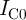and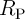, as the computations can be generalized by normalizing all current and resistance values to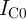and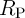, respectively. Furthermore, the effect of the pulse durations (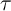) is negligible as compared to the internal exponential term in Eq. 4.15.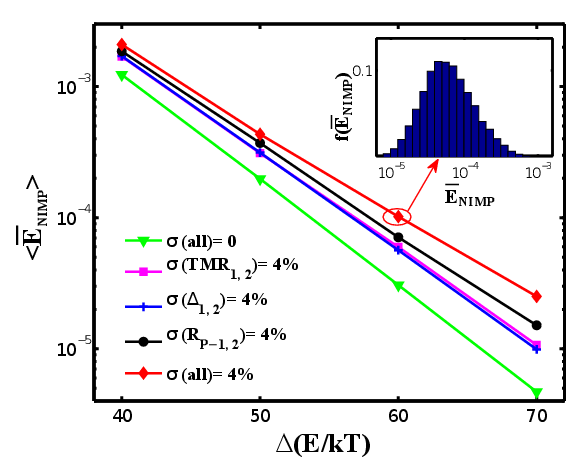Figure 4.25.: Expectation values of the NIMP error probability (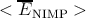) as a function of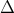.

In order to investigate the effect of the MTJ device-to-device variations, Fig. 4.25 shows the expectation value for the NIMP error probabilities (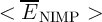) as a function of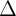under MTJ device variations with Gaussian distribution . The spread of the variations () is assumed to be 4% in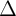, TMR ratio and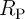for both target and source MTJs (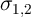). The mean values (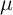) for TMR ratio and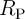are equal to 250% and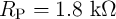, respectively. For each point, circuit parameters are fixed to the values which minimize the error and the average error probability for all combinations of input states (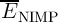) with 10000 random variations is calculated. Then the expectation error values are obtained by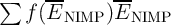, where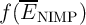is the distribution function of the errors (shown inset in Fig. 4.25). As it is expected, MTJ device variations increase the error probabilities as shown in Fig. 4.25.